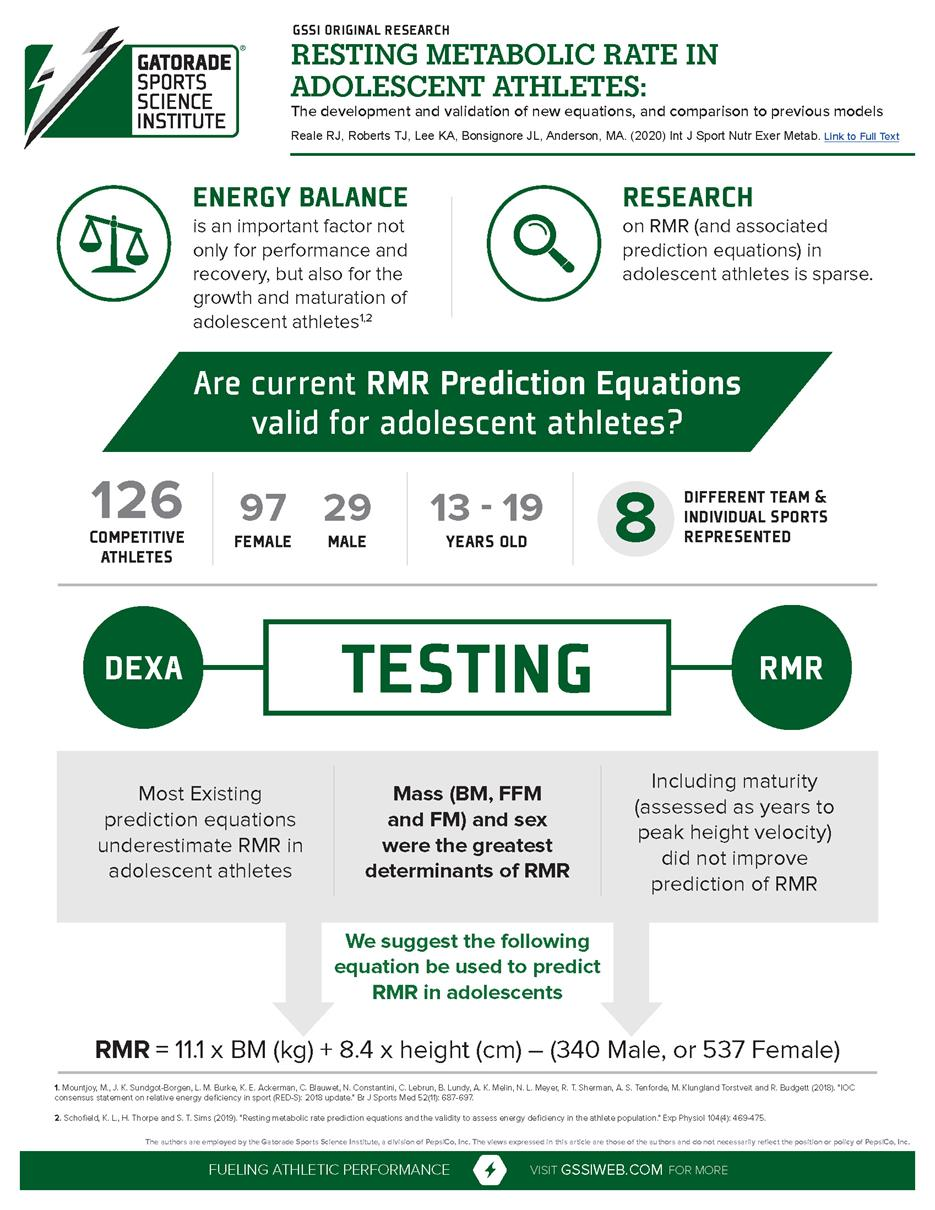# Resting metabolic rate in adolescent athletes: the development and validation of new equations, and comparison to previous models

Published

May 2020

Topics

## Abstract

We sought to assess the accuracy of current or developing new prediction equations for resting metabolic rate (RMR) in adolescent athletes. RMR was assessed via indirect calorimetry, alongside known predictors (body composition via dual-energy X-ray absorptiometry, height, age, and sex) and hypothesized predictors (race and maturation status assessed via years to peak height velocity), in a diverse cohort of adolescent athletes (n = 126, 77% male, body mass = 72.8 ± 16.6 kg, height = 176.2 ± 10.5 cm, age = 16.5 ± 1.4 years). Predictive equations were produced and cross-validated using repeated k-fold cross-validation by stepwise multiple linear regression (10 folds, 100 repeats). Performance of the developed equations was compared with several published equations. Seven of the eight published equations examined performed poorly, underestimating RMR in >75% to >90% of cases. Root mean square error of the six equations ranged from 176 to 373, mean absolute error ranged from 115 to 373 kcal, and mean absolute error SD ranged from 103 to 185 kcal. Only the Schofield equation performed reasonably well, underestimating RMR in 51% of cases. A one- and two-compartment model were developed, both r2 of .83, root mean square error of 147, and mean absolute error of 114 ± 26 and 117 ± 25 kcal for the one- and two-compartment model, respectively. Based on the models' performance, as well as visual inspection of residual plots, the following model predicts RMR in adolescent athletes with better precision than previous models; RMR = 11.1 × body mass (kg) + 8.4 × height (cm) - (340 male or 537 female).